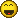# Break Out Indicator250

Hi all,

Hope all here are doing good and i need to code a simple indicator to test a break out strategy and below i explained simple logic then need to be coded as indicator

1. Allow user to use custom input for number of bar need to be computed for indicator calculation.

2. Calculate the Highest High HH and Lowest Low LL of above input period.

3. Generate signals when current price breaks HH LL of input period ( Signal as Arrow prefered )

5. Calculating targets use factor 4x ( not forex) of input period used above. For eg : if input is 3 period used then calculate 12 period HH LL as target plot ( Dots prefered )

6. Use opposite of HH LL value as stop point

I hope some one help me to code and test this wonderful indicator. GLGT to all